# Electronic structure of NiO with DFT+U¶

Version: 2017

By tuning the empirical Hubbard parameter U, one can sometimes obtain the correct band gap for semiconductors even with LDA or GGA. This tutorial shows how to approach this type of calculations by taking NiO as an example, and at the same time it also introduces the density of states (DOS) functionality in QuantumATK.

## Introduction¶

The self-interaction error is probably the most serious drawback of the LDA and GGA approximations to the exchange-correlation energy. This self-interaction can be described as the spurious interaction of an electron with itself. It has two main consequences:

• Electrons are over-delocalized;
• Band gaps in semiconductors and insulators are predicted to be much lower than their real counterpart.

The mean-field Hubbard correction, popularly called DFT+U, is a semi-empirical correction which tries to improve on these deficiencies.

In the DFT+U an additional energy term,

$E_{U} = \frac{1}{2} \sum_\mu U_\mu \left( n_\mu - n^2_\mu \right),$

is added to the Exchange-correlation energy [CdG05]. In this equation, $$n_\mu$$ is the projection onto an atomic shell and $$U_\mu$$ is the value of the “Hubbard U” for that shell. The $$E_{U}$$ energy term is zero for a fully occupied or unoccupied shell, while positive for a fractionally occupied shell.

The energy is thereby lowered if states become fully occupied. This may happen if the energy levels move away from the Fermi Level, i.e. increasing the band gap, or if the broadening of the states is decreased, i.e. the electrons are localized. In this way, the Hubbard U improves on the deficiencies of LDA and GGA.

The NiO crystal has a too low band gap in LDA and GGA and is one of the standard examples of how the DFT+U approximation can be used to improve the description of the electronic structure of solids [SLP99]. In this tutorial you will compare the DFT and DFT+U models for this system using the GGA.

Further details of the Hubbard U implementation in QuantumATK can be found in the ATK Reference Manual, particularly the section XC+U mean-field Hubbard term.

## The electronic structure of NiO calculated with DFT¶

NiO has a fcc crystal structure with two atoms in the unit cell. The Ni atoms have a net magnetic moment and form an anti-ferromagnetic arrangement in the (111) direction of the fcc cell. The structure can be described by a rhombohedral unit cell with 4 atoms in the basis [CdG05]. The structure is given below in the QuantumATK ATK-Python format:

# Set up lattice
lattice = Rhombohedral(5.138*Angstrom, 33.5573*Degrees)

# Define elements
elements = [Nickel, Oxygen, Nickel, Oxygen]

# Define coordinates
fractional_coordinates = [[ 0.  ,  0.  ,  0.  ],
[ 0.25,  0.25,  0.25],
[ 0.5 ,  0.5 ,  0.5 ],
[ 0.75,  0.75,  0.75]]

# Set up configuration
bulk_configuration = BulkConfiguration(
bravais_lattice=lattice,
elements=elements,
fractional_coordinates=fractional_coordinates
)


Copy the script and save it as NiO.py, or download it directly: NiO.py.

Note

The rhombohedral unit cell vectors are given as $$( 1,\frac{1}{2},\frac{1}{2}) a$$, where $$a$$ is the fcc lattice constant. The length of the rhombohedral unit cell vectors are therefore given by $$\sqrt{\frac{3}{2}} a$$, and are in accordance with the experimental fcc lattice constant of 4.19 Å.

### Setting up the calculation¶

You will in this section set up a spin-polarized DFT calculation using the spin-polarized version of the generalized gradient approximation (SGGA) for the NiO electronic structure and calculate the Mulliken population and density of states. If you are not familiar with the workflow of QuantumATK you are recommended to first go through the Basic QuantumATK Tutorial.

Start up QuantumATK and drag the script NiO.py onto theBuilder. The NiO crystal will be added to the Stash.Next, click the the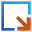button and select the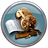Script Generator.

Tip

Alternatively you can drag the script NiO.py directly onto theScripter from the QuantumATK Project Files list.

Change the default output file name to NiO_sgga.hdf5 and add the following blocks to the script:Now double-click theNew Calculator to open the calculator widget, and set the k-points sampling to 6x6x6.Note

When an even sampling grid is used, the grid is automatically shifted to make sure that the $$\Gamma$$-point is included. The automatic shift can be avoided by unticking the option “Shift to $$\Gamma$$”.

The next step is to set up the anti-ferromagnetic NiO spin arrangement. For this purpose, open the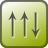Initial State block.

Select User spin – this will allow you to individually set the spin on each atom. Set opposite spins on the two nickel atoms and no spin on the oxygen atoms as illustrated below.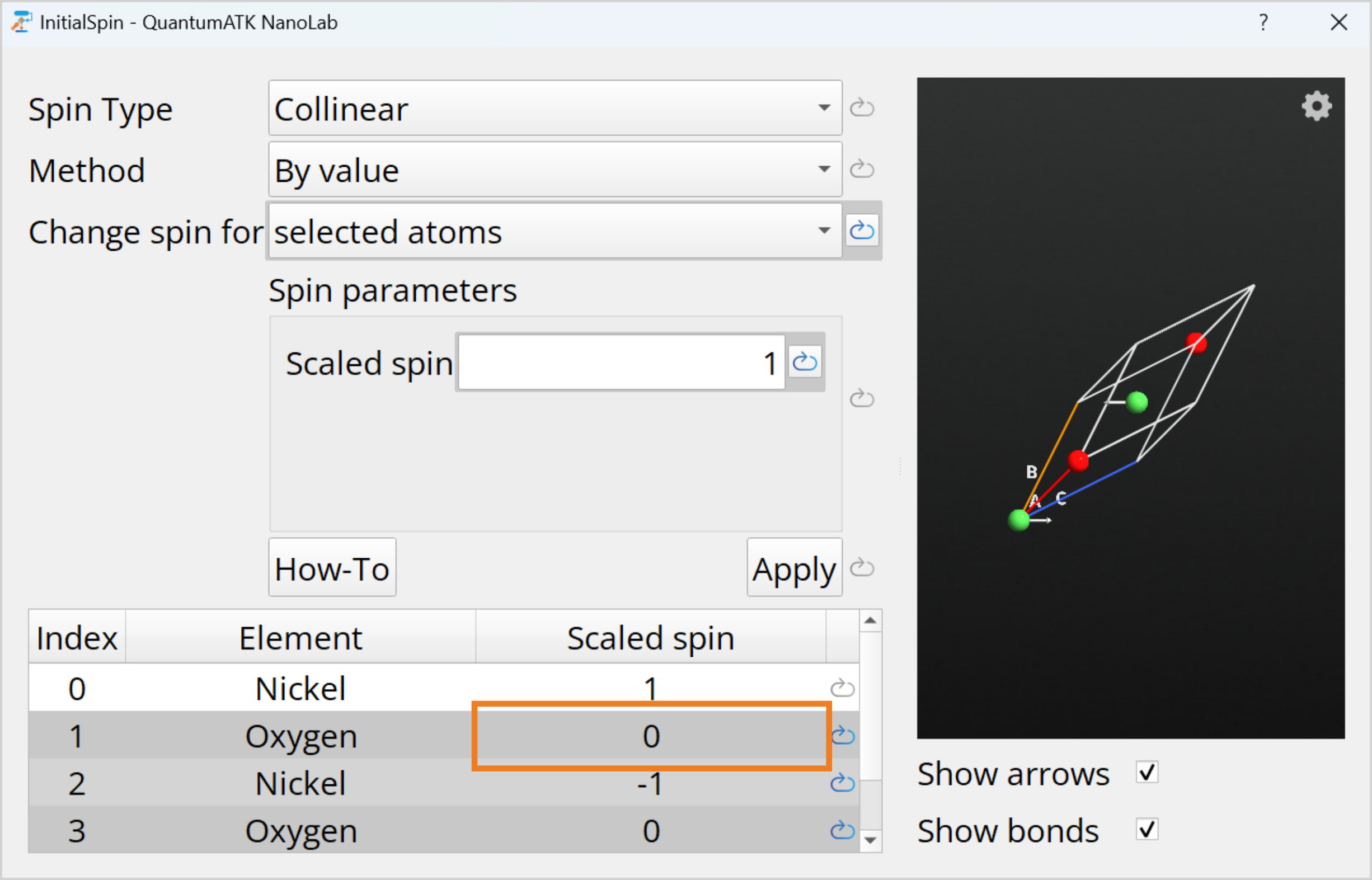Important

The initial spin on each atom is given relative to the atomic spin of the element as obtained by Hund’s rule. For nickel the electronic configuration of the atom is [Ar]3d84s2 (see periodic table in the ATK Reference Manual.). The 3d shell is fractionally occupied, and only this shell will contribute to the spin of the atom. According to Hund’s rule the 3d shell has 5 electrons in the up direction and 3 electrons in the down direction, giving a total atomic spin of 2$$\mu_B$$ for nickel.

Finally, open theDensityOfStates block and set the k-points sampling to 10x10x10. In general, one should choose a quite dense k-points grid for DOS analyses, in order to capture possible sharp features in the density of states.Save the script as NiO_sgga.py, but do not close the Scripter window – you will need it again later.

### Performing the calculation¶

To start the calculations, send the script to the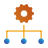Job Manager by using thebutton.

Choose a Local machine for executing the job and clickstart. The job will finish after 1-2 minutes and you can inspect the results.

### Analysing the results¶

#### Mulliken Population¶

To inspect the Mulliken population reported in the calculation log file, scroll down to the end of the log file and you will find a report as shown below.

 6605 6606 6607 6608 6609 6610 6611 6612 6613 6614 6615 6616 6617 6618 6619 6620 6621 6622 6623 6624 6625 6626 6627 6628 6629 6630 6631 6632 6633 6634 +------------------------------------------------------------------------------+ | | | Mulliken Population Report | | | | ---------------------------------------------------------------------------- | | | | | Element Total Shell | Orbitals | | | | | | s | | 0 Ni 9.244 0.994 | 0.994 | | 7.892 0.994 | 0.994 | | | y z x | | 2.997 | 0.999 0.999 0.999 | | 2.996 | 0.999 0.999 0.999 | | | s | | 0.127 | 0.127 | | 0.127 | 0.127 | | | xy zy zz-rr zx xx-yy | | 4.887 | 0.986 0.986 0.964 0.986 0.964 | | 3.533 | 0.980 0.980 0.297 0.980 0.297 | | | y z x | | 0.042 | 0.014 0.014 0.014 | | 0.044 | 0.015 0.015 0.015 | | | xy zy zz-rr zx xx-yy | | 0.056 | 0.007 0.007 0.018 0.007 0.018 | | 0.053 | 0.007 0.007 0.016 0.007 0.016 | | | y z x | | 0.140 | 0.047 0.047 0.047 | | 0.145 | 0.048 0.048 0.048 | | | --------------------------------------------------- | 

Tip

The Mulliken population can also be inspected by selecting theMulliken Population item on the QuantumATK LabFloor. Use the Text Representation tool in the right-hand panel to see the results.

The Mulliken population reports the numbers of electrons per spin and orbital, as well as the orbital sum for each atom. Note that oxygen atoms are not polarized while the two nickel atoms are polarized in opposite directions, thus forming an anti-ferromagnetic arrangement. The polarization can be calculated from the difference between the number of electrons in the spin-up channel (9.244) and that in the spin-down channel (7.892). The resulting value of 1.35$$\mu_B$$ is in good agreement with other DFT calculations [CdG05].

#### Projected density of states¶

To investigate the NiO density of states (PDOS), select theDensityOfStates item on the LabFloor, click the 2D Plot tool in the right-hand panel. You may need to zoom in a little on the plot; use the left mouse button for this.Tip

The plot shows the total density of states of the spin-up channel with a black line and minus the result for the spin-down channel with a red line. If you de-select Flip spin-down in the Options menu, the density of states of the spin-down channel will also be plotted on the positive axis.

The total DOS shows no difference between the two spin channels. However, you saw from the Mulliken population that the nickel atoms are spin polarized!

To inspect the projected DOS corresponding to just one nickel atom, select a nickel atom with the left-hand button of the mouse, as illustrated below.The PDOS is simply the total DOS projected onto the selected nickel atom. The expected difference between the spin-up and spin-down DOS channels is now apparent.

Tip

You can also create combined projections by selecting multiple atoms (use the left-hand button of the mouse while holding Ctrl) and more than one shell.

The calculation predicts a band gap of ~0.8 eV, which is much smaller than the experimental value of 4.0 eV [AAL97]. In the next chapter you will see how the description of the band gap is improved with the DFT+U approximation.

## DFT+U calculation for the NiO crystal¶

You will now perform a DFT+U calculation of the NiO crystal, using U = 4.6 eV for the nickel d-states, as proposed in [CdG05].

### Calculations¶

You will need to modify the script generated above for the SGGA calculation. Open theScripter used in the previous chapter and make the following modifications:

• Change the default output file name to NiO_sgga_u.nc.
• Change the Script detail to Show defaults.Open theNew Calculator:

• Switch to the Basis set/exchange correlation tab.
• Change the option Hubbard U from Disabled to Onsite.
• In the Basis set section, click Set in the column on the right-hand side on the row corresponding to nickel, and set the Hubbard U parameter for the 3d orbital to 4.6 eV.Next transfer the script to the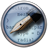Editor using the send tobutton, in order to check that all the parameters are properly set.

In the editor locate the line where the nickel basis is defined and check that the hubbard U parameter is set to 4.6 eV for the nickel_3d and nickel_3d_0 orbitals.

 190 191 192 193 194 195 196 197 198 199 NickelBasis = BasisSet( element=PeriodicTable.Nickel, orbitals=[nickel_3s, nickel_3p, nickel_4s, nickel_3d, nickel_3p_0, nickel_3d_0, nickel_4p], occupations=[2.0, 6.0, 0.0, 8.0, 1.0, 1.0, 0.0], hubbard_u=[0.0, 0.0, 0.0, 4.6, 0.0, 4.6, 0.0]*eV, dft_half_parameters=Automatic, filling_method=SphericalSymmetric, onsite_spin_orbit_split=[0.0, 0.0, 0.0, 0.0, 0.0, 0.0, 0.0]*eV, pseudopotential=NormConservingPseudoPotential("normconserving/sg15/gga/28_Ni.upf"), ) 

Now save the script as NiO_sgga_u.py and execute the job using theJob Manager.

### Analyzing the results¶

First inspect the Mulliken population in the log file. You should find a magnetic moment of 1.80$$\mu_B$$ on a nickel atom, which is in good agreement with the experimental result of 1.64 – 1.9$$\mu_B$$ [AAL97].

To determine the band gap, inspect the printed density of states in the log file. You should find that the DOS is zero in the range -1.69 to 1.69 eV, corresponding to a band gap of 3.38 eV. This is much higher than the SGGA value of 0.8 eV, and in better agreement with the experimental value of 4.0 eV [AAL97].

#### Comparing the DFT and DFT+U projected DOS¶

The final step is to compare the nickel PDOS obtained with DFT and DFT+U. You will here use Python scripting to perform the analysis.

The script dos-comparision.py performs the analysis. It is shown below:

  1 2 3 4 5 6 7 8 9 10 11 12 13 14 15 16 17 18 19 20 21 22 23 24 25 26 27 28 29 30 31 32 33 34 35 36 37 38 39 #read in the dos object dos = nlread('NiO_lsda.hdf5',DensityOfStates) #generate some energies energies = numpy.linspace(-5,5,400)*eV #calculate the spectrum n0_up = dos.tetrahedronSpectrum(energies=energies, spin=Spin.Up, projection_list = ProjectionList()) n0_down = dos.tetrahedronSpectrum(energies=energies, spin=Spin.Down, projection_list = ProjectionList()) e = dos.energies() #do the same for LSDA+U dos_u = nlread('NiO_lsda_u.hdf5',DensityOfStates) n0_up_u = dos_u.tetrahedronSpectrum(energies=energies, spin=Spin.Up, projection_list = ProjectionList()) n0_down_u = dos_u.tetrahedronSpectrum(energies=energies, spin=Spin.Down, projection_list = ProjectionList()) #plot the spectrum using pylab import pylab #first plot the up component with dots pylab.plot(e.inUnitsOf(eV), n0_up.inUnitsOf(eV**-1), 'k:',label = 'SGGA') #now plot the down component with negative values and dots pylab.plot(e.inUnitsOf(eV), -1.*n0_down.inUnitsOf(eV**-1), 'k:') #now plot the LSDA+U up components with solid pylab.plot(e.inUnitsOf(eV), n0_up_u.inUnitsOf(eV**-1),'k',label = 'SGGA+U') #now plot the LSDA+U down component with negative values and solid pylab.plot(e.inUnitsOf(eV), -1.*n0_down_u.inUnitsOf(eV**-1),'k') #show legends pylab.legend() pylab.xlabel("Energy (eV)") pylab.ylabel("DOS (1/eV)") pylab.show() 

Download the script and execute it using theJob Manager. The following plot is produced, illustrating the projected DOS for the nickel atom obtained using SGGA and SGGA+U. Notice the large difference in band gap between the two calculations (region of zero DOS around the Fermi level, at 0 eV energy).Note

The plotting is based on the matplotlib package which is part of QuantumATK, see Plotting using pylab for more information.